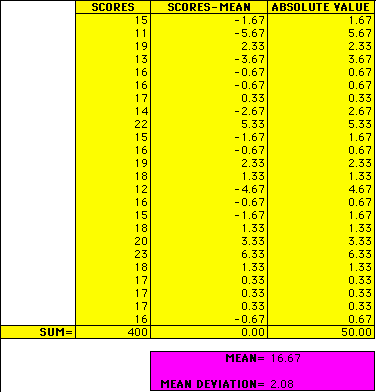Do Deviation Scores Have Meaning?... Absolutely!

Take your dice scores and find the mean. Next subtract the mean from each score to find the deviation score. Take the absolute value of the deviation scores and then add them all up.

HINT: A spreadsheet would be very helpful here and you can download one if you have access to Excel 3.0 or some other compatible program.

Once you have taken the absolute value of all the deviation scores and added them all up, divide the sum by the number of scores. This will give you the average distance of the observed scores from the mean.

Take a look at these sample scores:How is this measure useful?

Now let's take a look at what happens when we square the deviation scores rather than taking the absolute value, or if you have already studied variance, you may skip to the end.